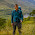Wednesday, January 1, 2014

SPOJ HOLI

Given a weighted tree, consider there are N people in N nodes. You have to rearrange these N people such that everyone is in a new node, and no node contains more than one people and maximizes the cost. Here cost is the distance travelled for each person.

First observation is, in order to maximize cost, all edges will be used to travel around. So, if we can calculate how many times each edge will be used, we can calculate the cost.

Now for any edge Ei, we can partition the whole tree into two subtrees, if one side has n nodes, the other side will have N - n nodes. Also, note that, min(n, N-n) people will be crossing the edge from each side. Because if more people cross the edge, then by pigeon-hole principle in one side, we will get more people than available node which is not allowed in the problem statement. So, Ei will be used a total of 2 * min(n, N-n) times.

Thus the final result is:

`cost = ∑ 2*min(ni, N-ni)*wieght(Ei) | for each edge Ei`

Here, ni is the count for nodes in one side of the edge Ei and N-ni on the other side. So, we can use simple DFS algorithm to solve the problem. During the traversal, just count number of nodes in the subtree for an edge u⇒v and calculate result for each edge in the process.

1 comment:

1.Really beautiful application of Pigeonhole Principle.